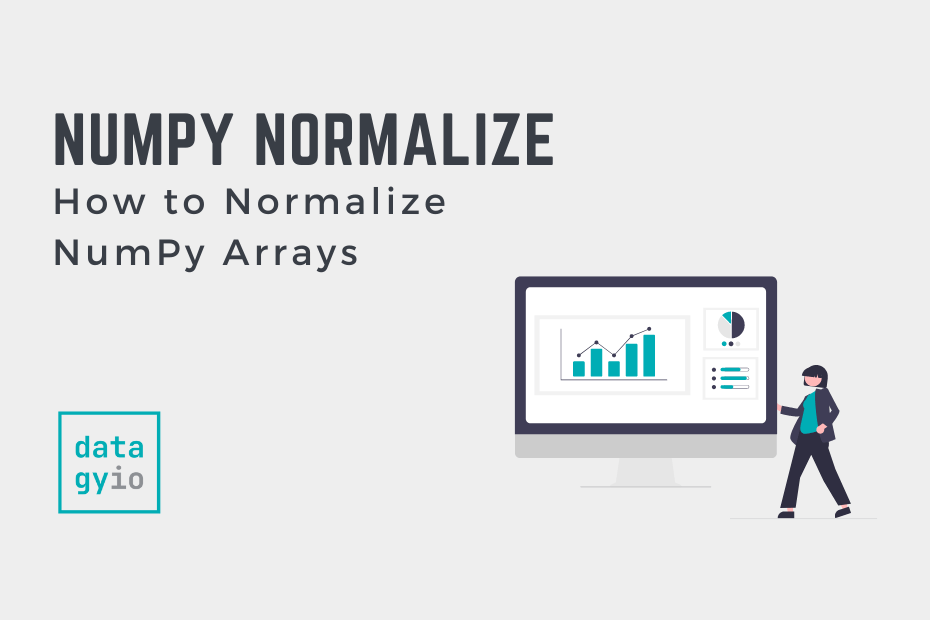# Python## How to Normalize NumPy Arrays (Min-Max Scaling, Z-Score, L2)

In this tutorial, you’ll learn how normalize NumPy arrays, including multi-dimensional arrays. Normalization is an important skill for any data analyst or data scientist. Normalization refers to the process of scaling data within a specific range or distribution to make… Read More »How to Normalize NumPy Arrays (Min-Max Scaling, Z-Score, L2)## Pandas GroupBy Multiple Columns Explained with Examples

The Pandas groupby method is a powerful tool that allows you to aggregate data using a simple syntax, while abstracting away complex calculations. One of the strongest benefits of the groupby method is the ability to group by multiple columns,… Read More »Pandas GroupBy Multiple Columns Explained with Examples## Pandas Drop a Dataframe Index Column: Guide with Examples

Learn how to use Pandas to drop a dataframe index column using the reset_index and set_index methods and how to read csv without an index.## Pandas Quantile: Calculate Percentiles of a Dataframe

Learn how to use the Pandas quantile method to calculate percentiles in Pandas including how to modify the interpolation of values.## Python strptime: Converting Strings to DateTime

Learn how to convert a Python string to date using the datetime module’s strptime function. Also learn how to do this to a Pandas dataframe!## Python strip: How to Trim a String in Python

In this tutorial, we’ll dive into the world of Python string trimming! String trimming is an essential skill that helps you clean up and refine your text data, making it more accurate and easier to work with. For example, being… Read More »Python strip: How to Trim a String in Python## How to Calculate a Rolling Average (Mean) in Pandas

In this post, you’ll learn how to calculate a rolling mean in Pandas using the rolling() function. Rolling averages are also known as moving averages. Creating a rolling average allows you to “smooth” out small fluctuations in datasets, while gaining insight into trends.… Read More »How to Calculate a Rolling Average (Mean) in Pandas## Pandas unique(): Get Unique Values in a DataFrame

Pandas provides a lot of different ways to interact with unique values. Learn how to get unique values as a list, get unique values across columns and more!## Unpivot Your Data with the Pandas Melt Function

You can easily unpivot and reshape data you with python by using Pandas and the Melt function! Find out how using this thorough overview!## Pandas date_range: How to Create a Date Range in Pandas

In this tutorial, we’re diving deep into one of the essential and versatile tools of the Pandas library—the date_range function. Whether you’re a beginner just starting to explore the power of Pandas or already an adept user, this function is… Read More »Pandas date_range: How to Create a Date Range in Pandas## Calculating Standard Deviation in Python: A Comprehensive Guide

In Python, Standard Deviation can be calculated in many ways – learn to use Python Statistics, Numpy’s, and Pandas’ standard deviant (std) function.## Pandas Reset Index: How to Reset a Pandas Index

Learn how to use the Pandas reset index method to reset an index, including working with a multi-index and dropping the original index.